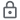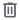# Equation Solver Calculators

10K+Everyone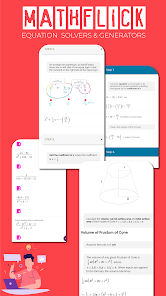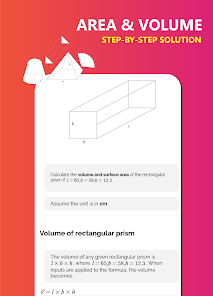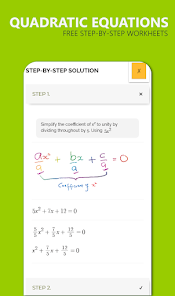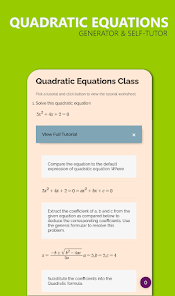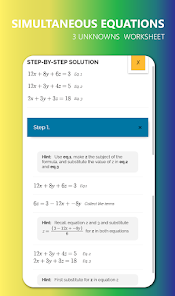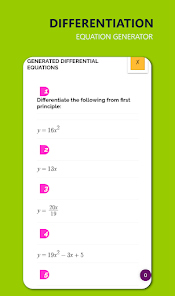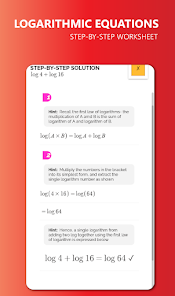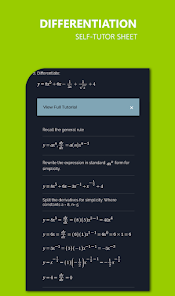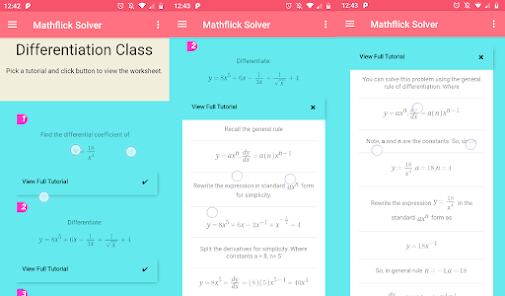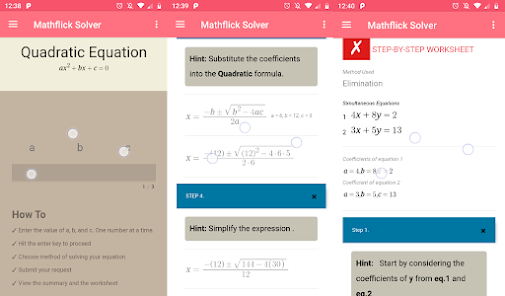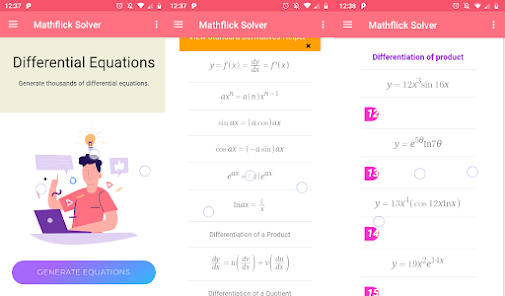Math equation solver with step by step solutions. Mathflick is a math solving app that solves and generates thousands of equations with complete step by step solutions on quadratic equation, simultaneous equations, logarithmic, partial fractions, linear equations, differential equation, number sequence and inequalities.

Mathflick math app comes with a rich compilation of step-by-step worksheet that solves and generate maths equations on a fly.

Included in the updated versions are - bodmas game, debt to income calculator, mortgage calculator, engineering unit conversion, equation generators, graph plotter and more equation solver that solves differential equation, linear equation and logarithms.

⥭ What It Does

✓ Generate Unlimited Math Equations
✓ Solve Math Problems Step By Step
✓ Calculator and Graph Plotter
✓ Math Quiz, questions and answers
✓ Math Equation Solver
✓ Interactive Bodmas Game

⥭ Math Equation Solver Included

█ Simultaneous Equation Solver

✓ Solves simultaneous equation of 2 unknowns in this form:
x + y = 10,
x - y = 12

✓ Solves simultaneous equation of 3 unknowns in this form
Equation 1 x +y + z = 16
Equation 2 x +y + z = 8
Equation 3 x +y + z = 9

You can solve any quadratic equations by
✓ Factorization method
✓ Completing the square

█ Area and Volume of Shape Problem Solver
✓ Area, perimeter and diagonal of Square
✓ Area, perimeter and diagonal of Rectangle
✓ Area and perimeter of Parallelogram
✓ Area of Triangle
✓ Area, perimeter and diagonal of Trapezium
✓ Area and circumference of circle
✓ Area of Sector in radians and degrees
✓ Volume, curved surface and total surface area of Cylinder
✓ Volume, curved surface and total surface area of Cone
✓ Area and perimeter of Ellipse
✓ Volume of Triangular Prism
✓ Volume and surface area of Rectangular Prism
✓ Volume, curved surface and total surface of Frustum of Cone

█ Linear Equation Solver
✓ Solves linear equation in five different format

█ Logarithmic Solver
✓ Solve logarithms using all the basic rules.

█ Equation Generators (Teaching Tool)
Mathflick equation generator generates the following set of equations on

✓ Partial Fraction
✓ Simultaneous Equation
✓ Differentiation
✓ Linear Equation
✓ Logarithms
✓ Number Sequence
✓ Inequalities

█ Bodmas Game(Fun Tool)
Mathflick equation generator generates the following set of equations on

█ Calculator(Utility)
✓ Income to Debt Ratio Calculator
✓ Mortgage Calculator
✓ Engineering Unit Converter
█ Graph (Utility)
✓ Linear Equation Graph Plotter
✓ Laplace, differentiation, integration graph plotter
Updated on
Jan 20, 2022

## Data safety

Safety starts with understanding how developers collect and share your data. Data privacy and security practices may vary based on your use, region, and age. The developer provided this information and may update it over time.This app may share these data types with third parties
Location, Personal info and 2 othersNo data collected